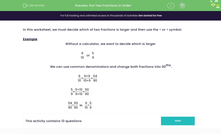# Compare the Size of Two Fractions

In this worksheet, students will decide which of two fractions is larger by using common denominators to compare their sizes.Key stage:  KS 3

Curriculum topic:   Number

Curriculum subtopic:   Understand Order for All Numbers

Difficulty level:#### Worksheet Overview

In this activity, we will decide which of two fractions is larger and then use the < or > symbol.

Example

Without a calculator, we need to decide which is larger.

6/10    or    5/9

We need to find the lowest common multiple of 10 and 9 to create common denominators and change both fractions into 90ths

Starting with 6/10, we multiply 10 by 9 to get 90 as the denominator.

We have to do the same thing to the numerator, so we multiply 6 by 9 too, to get 54.

So, 6/10 has now become 54/90

Now to 5/9

We multiply the denominator of 9 by 10 to get 90.

We need to do the same to the numerator of 5, so 5 x 10 gives us 50.

So, 5/9 has become 50/90

We now have 54/90 and 50/90

54/90  > 50/90, so 6/10 >  5/9Let's have a go at some questions now.

### What is EdPlace?

We're your National Curriculum aligned online education content provider helping each child succeed in English, maths and science from year 1 to GCSE. With an EdPlace account you’ll be able to track and measure progress, helping each child achieve their best. We build confidence and attainment by personalising each child’s learning at a level that suits them.

Get started••••